### Semiconductor Electronics: Materials, Devices And Simple Circuits Class 12th Physics Part Ii CBSE Solution

##### Question 1.The number of silicon atoms per m3 is 5 × 1028. This is doped simultaneously with 5 × 1022 atoms per m3 of Arsenic and 5 × 1020 per m3 atoms of Indium. Calculate the number of electrons and holes. Given that ni = 1.5 × 1016 m–3. Is the material n-type or p-type?Answer:Given,Number of Silicon atoms per m3 = 5 × 1028Number of Arsenic atoms per m3 (nAs) = 5 × 1022Number of Indium atoms per m3 (nIn) = 5 × 1020Intrinsic charge carrier concentration (ni) = 1.5 × 1016 m-3So, number of electrons per m-3 (ne) = nAs – nii.e., ne = 5 × 1022 – 1.5 × 1016 = 4.99 × 1022 m-3If nh is the number of holes per m-3, then according to Mass-action law,ni2 = nenhSo, nh = ni2/nei.e., nh =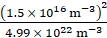So, nh= 4.5 × 109 m-3The number of electrons is higher than the number of holes. So, the given material is n-type.NOTE: Arsenic is pentavalent and Indium is trivalent. In an n-type semiconductor, electrons are the majority charge carriers i.e., ne≫nh. In a p-type semiconductor, holes are the majority charge carriers i.e., nh≫ne.Question 2.In an intrinsic semiconductor, the energy gap Eg is 1.2eV. Its hole mobility is much smaller than electron mobility and independent of temperature. What is the ratio between conductivity at 600K and that at 300K? Assume that the temperature dependence of intrinsic carrier concentration ni is given by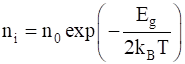where n0 is a constant.Answer:Given,Energy gap (Eg) = 1.2eVThe temperature dependence of intrinsic carrier concentration is given asWhere T is the temperatureEg is the forbidden energy gapKB is the Boltzmann’s constant (8.62 × 10-5 eV/K)no is a constant.At temperature T1 = 600K,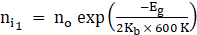……….(1)At temperature T2 = 300K,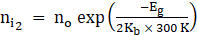…..(2)Dividing eqn. (1) by eqn. (2), we get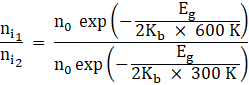⇒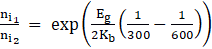⇒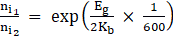⇒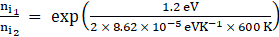⇒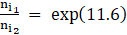So,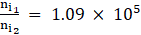Hence, the ratio of conductivity at 600K to that at 300K is 1.09 × 105.NOTE: 1. Eg is the forbidden energy gap which is the energy gap between the valence band and conduction bands.2. Intrinsic semiconductors are pure semiconductors with fewer conductivities. Trivalent and pentavalent impurities are added to intrinsic semiconductors to form p-type and n-type semiconductors respectively. This process is called doping.Question 3.In a p-n junction diode, the current I can be expressed as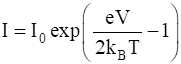Where I0 is called the reverse saturation current, V is the voltage across the diode and is positive for forward bias and negative for reverse bias, and I is the current through the diode, kB is the Boltzmann constant (8.6 × 10–5 eV/K) and T is the absolute temperature. If for a given diode I0 = 5 × 10–12 A and T = 300 K, then(a) What will be the forward current at a forward voltage of 0.6 V?(b) What will be the increase in the current if the voltage across the diode is increased to 0.7 V?(c) What is the dynamic resistance?(d) What will be the current if reverse bias voltage changes from 1 V to 2 V?Answer:Given,Reverse saturation current (Io) = 5 × 10-12 ATemperature (T) = 300KThe diode current (I) is given by the relation,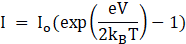Where Io is the reverse saturation currentV is the voltage across the diodekB is the Boltzmann’s constantT is the absolute temperaturee is the electronic charge (1.6 × 10-19 C)Note: The value of kB (in electron volts) is 8.6 × 10–5 eV/K and also 1.38 × 10-23 kg m2s-2K-1)(a) For V = 0.6V,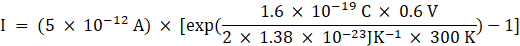⇒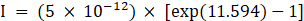⇒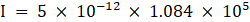So, I = 5.42 × 10-7 A(b) For V = 0.7V,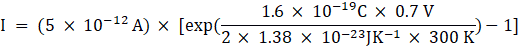⇒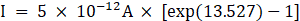⇒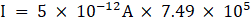So, I = 3.74 × 10-6 AHence, increase in current = 3.74 × 10-6 - 5.42 × 10-7 = 3.198 × 10-6 A(c)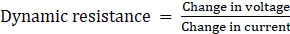So, dynamic resistance =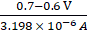i.e., Dynamic resistance = 31269.5 Ω = 31.26kΩ(d) If the reverse bias voltage changes from 1V to 2V, then the diode current remains equal to reverse saturation current. So, dynamic resistance will be infinite.NOTE: A diode is said to be in forward bias if the p-side and n-side are connected to the positive and negative terminals of the battery respectively. Electric current flowing through the diode in this condition is known as forward diode current. If the p-side is connected to the negative terminal and n-side is connected to the positive terminal of the battery, then the diode is said to be in reverse bias.Question 4.You are given the two circuits as shown in Fig. 14.44. Show that circuit (a) acts as OR gate while the circuit (b) acts as AND gate.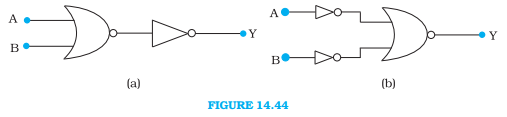Answer:The circuit (a) is as follows: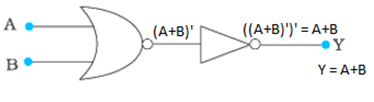STEPS:1. The output of the NOR gate is the complement of A + B i.e., (A + B)’.2. The NOT gate gives the complement of this output.3. So, the output Y = A + B.The truth table for this circuit is as follows:The circuit (b) is as follows: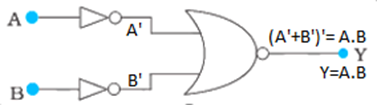STEPS:1. The NOT gates give the complements of the inputs A and B i.e., A’ and B’.2. The NOR gate gives the output as (A’ + B’)’.3. Using De Morgan’s law, (A’ + B’)’ = ((A∙B)’)’ = A∙B4. So, the output Y = A∙B.Question 5.Write the truth table for a NAND gate connected as given in Fig. 14.45.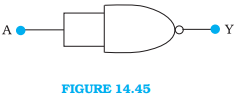Hence identify the exact logic operation carried out by this circuit.Answer:The output is obtained as (A∙A)’ = A’. (Since, A∙A = A)Hence, the given gate acts as a NOT gate.The truth table is given as follows:NOTE: NAND gate is a universal gate because it can be used to implement any Boolean function without using any other gates.Question 6.You are given two circuits as shown in Fig. 14.46, which consist of NAND gates. Identify the logic operation carried out by the two circuits.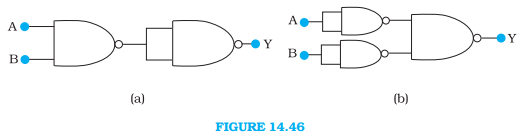Answer:The circuit (a) is as follows: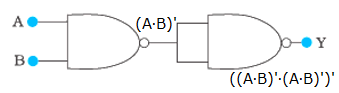The first NAND gate gives the output (A∙B)’. The second NAND gate gives the output ((A∙B)’∙(A∙B)’)’.Using De Morgan’s law,((A∙B)’∙(A∙B)’)’ = ((A∙B)’)’ + ((A∙B)’)’ = (A∙B) + (A∙B) = A∙B (Since, A + A = A)So, the output is Y = A∙B. The circuit acts as an AND gate.The truth table for the circuit is as follows:The circuit (b) is as follows: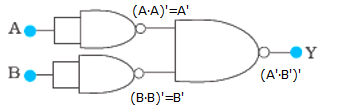The first NAND gate gives (A∙A)’ = A’ as the output. The second NAND gate gives (B∙B)’ = B’ as the output. The last NAND gate gives (A’∙B’)’ as the output.Using De Morgan’s law,(A’∙B’)’ = (A’)’ + (B’)’ = A + BSo, the output is A + B. The circuit acts as an OR gate.The truth table for the circuit is as follows:NOTE: NAND gate is a universal gate because it can be used to implement any Boolean function without using any other gates.Question 7.Write the truth table for circuit given in Fig. 14.47 below consisting of NOR gates and identify the logic operation (OR, AND, NOT) which this circuit is performing.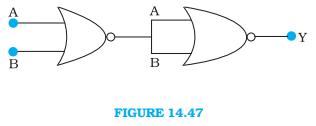(Hint: A = 0, B = 1 then A and B inputs of second NOR gate will be 0 and hence Y = 1. Similarly, work out the values of Y for other combinations of A and B. Compare with the truth table of OR, AND, NOT gates and find the correct one.)Answer:The given circuit is as follows: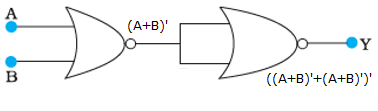The first NOR gate gives the output (A + B)’. The second NOR gate gives the output ((A + B)’ + (A + B)’)’.Using De Morgan’s law,((A + B)’ + (A + B)’)’ = ((A + B)’)’∙((A + B)’)’ = (A + B)∙(A + B) = A + B (Since, A∙A = A)So, the output is Y = A + B and the circuit acts as an OR gate.The truth table for the given circuit is as follows:NOTE: NOR gate is a universal gate because it can be used to implement any Boolean function without using any other gates.Question 8.Write the truth table for the circuits given in Fig. 14.48 consisting of NOR gates only. Identify the logic operations (OR, AND, NOT) performed by the two circuits.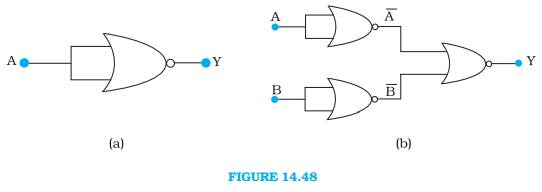Answer:The circuit (a) is as follows: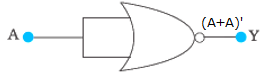The circuit gives the output (A + A)’ = A’.So, the output is Y = A’ and the circuit acts as a NOT gate.The truth table for the circuit is as follows:The circuit (b) is as follows: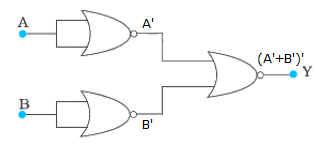Similar to the circuit (a), the first two gates give inverted output as A’ and B’. The third NOR gate gives the output (A’ + B’)’.Using De Morgan’s law,(A’ + B’)’ = (A’)’∙(B’)’ = A∙B (Since, (A’)’ = A)Hence, the output is Y = A∙B and the circuit acts as an AND gate.The truth table is as follows:NOTE: NOR gate is a universal gate because it can be used to implement any Boolean function without using any other gates.

PDF FILE TO YOUR EMAIL IMMEDIATELY PURCHASE NOTES & PAPER SOLUTION. @ Rs. 50/- each (GST extra)

HINDI ENTIRE PAPER SOLUTION

MARATHI PAPER SOLUTION

SSC MATHS I PAPER SOLUTION

SSC MATHS II PAPER SOLUTION

SSC SCIENCE I PAPER SOLUTION

SSC SCIENCE II PAPER SOLUTION

SSC ENGLISH PAPER SOLUTION

SSC & HSC ENGLISH WRITING SKILL

HSC ACCOUNTS NOTES

HSC OCM NOTES

HSC ECONOMICS NOTES

HSC SECRETARIAL PRACTICE NOTES

# 2019 Board Paper Solution

HSC ENGLISH SET A 2019 21st February, 2019

HSC ENGLISH SET B 2019 21st February, 2019

HSC ENGLISH SET C 2019 21st February, 2019

HSC ENGLISH SET D 2019 21st February, 2019

SECRETARIAL PRACTICE (S.P) 2019 25th February, 2019

HSC XII PHYSICS 2019 25th February, 2019

CHEMISTRY XII HSC SOLUTION 27th, February, 2019

OCM PAPER SOLUTION 2019 27th, February, 2019

HSC MATHS PAPER SOLUTION COMMERCE, 2nd March, 2019

HSC MATHS PAPER SOLUTION SCIENCE 2nd, March, 2019

SSC ENGLISH STD 10 5TH MARCH, 2019.

HSC XII ACCOUNTS 2019 6th March, 2019

HSC XII BIOLOGY 2019 6TH March, 2019

HSC XII ECONOMICS 9Th March 2019

SSC Maths I March 2019 Solution 10th Standard11th, March, 2019

SSC MATHS II MARCH 2019 SOLUTION 10TH STD.13th March, 2019

SSC SCIENCE I MARCH 2019 SOLUTION 10TH STD. 15th March, 2019.

SSC SCIENCE II MARCH 2019 SOLUTION 10TH STD. 18th March, 2019.

SSC SOCIAL SCIENCE I MARCH 2019 SOLUTION20th March, 2019

SSC SOCIAL SCIENCE II MARCH 2019 SOLUTION, 22nd March, 2019

XII CBSE - BOARD - MARCH - 2019 ENGLISH - QP + SOLUTIONS, 2nd March, 2019

# HSCMaharashtraBoardPapers2020

(Std 12th English Medium)

HSC ECONOMICS MARCH 2020

HSC OCM MARCH 2020

HSC ACCOUNTS MARCH 2020

HSC S.P. MARCH 2020

HSC ENGLISH MARCH 2020

HSC HINDI MARCH 2020

HSC MARATHI MARCH 2020

HSC MATHS MARCH 2020

# SSCMaharashtraBoardPapers2020

(Std 10th English Medium)

English MARCH 2020

HindI MARCH 2020

Hindi (Composite) MARCH 2020

Marathi MARCH 2020

Mathematics (Paper 1) MARCH 2020

Mathematics (Paper 2) MARCH 2020

Sanskrit MARCH 2020

Sanskrit (Composite) MARCH 2020

Science (Paper 1) MARCH 2020

Science (Paper 2)

Geography Model Set 1 2020-2021

MUST REMEMBER THINGS on the day of Exam

Are you prepared? for English Grammar in Board Exam.

Paper Presentation In Board Exam

How to Score Good Marks in SSC Board Exams

Tips To Score More Than 90% Marks In 12th Board Exam

How to write English exams?

How to prepare for board exam when less time is left

How to memorise what you learn for board exam

No. 1 Simple Hack, you can try out, in preparing for Board Exam

How to Study for CBSE Class 10 Board Exams Subject Wise Tips?

JEE Main 2020 Registration Process – Exam Pattern & Important Dates

NEET UG 2020 Registration Process Exam Pattern & Important Dates

How can One Prepare for two Competitive Exams at the same time?

8 Proven Tips to Handle Anxiety before Exams!